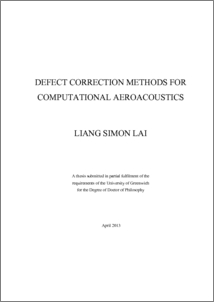# Defect correction methods for computational aeroacoustics

Lai, Liang Simon (2013) Defect correction methods for computational aeroacoustics. PhD thesis, University of Greenwich.Preview
PDF
Liang_Simon_Lai_2013.pdf - Published Version

## Abstract

The idea of Defect Correction Method (DCM) has been around for a long time. It can be used in a number of different ways and can be applied to solve various linear and non-linear problems. Most defect correction related methods were used in conjunction with discretisation methods and two-level multigrid methods. This thesis examines how various iterative methods, both for linear and nonlinear problems, may be built into a unified framework through the use of defect correction. The framework is extended to the area of Computational Aeroacoustics (CAA) where sound waves generated by the pressure fluctuations are typically several orders of magnitude smaller than the pressure variations in the main flow field that accounts for flow acceleration. A decomposition of variables is used to break down the components of a typical flow variable into (1) the mean flow, (2) flow perturbations or aerodynamic sources of sound, and (3) the acoustic perturbation. The framework as discussed in this thesis would incorporate such variable decomposition. The basic principle of DCM can be applied to recover the propagating acoustic perturbation through a coupling technique. This provides an excellent concept in the re-use of existing commercial CFD software based on the framework and in the retrieval of acoustic pressure. Numerical examples demonstrating the defect correction framework for a typical car sun-roof problem was examined with promising numerical results. To this end the complete process of coupling Reynolds average Navier-Stokes and the Helmholtz equation is also presented using the DCM framework.

The DCM framework is also extended to handle higher order numerical methods for the numerical solutions of partial differential equations leading to an easy re-use of existing software approximating derivatives with a lower order discretisation. Numerical experiments were performed to demonstrate the capability of the DCM framework. It is also used to a simplified 2-D problems aiming at the understanding of Large Eddy Simulation (LES) and filtering techniques. To this end the framework of DCM leads to an efficient and robust software implementation for many CFD and aeroacoustic computation in a simple nutshell.

Item Type: Thesis (PhD) uk.bl.ethos.601739 defect correction method (DCM), numerical analysis, Q Science > QA Mathematics School of Computing & Mathematical SciencesSchool of Computing & Mathematical Sciences > Department of Mathematical Sciences 17 Mar 2017 12:28 None None None None http://gala.gre.ac.uk/id/eprint/11452View Item